Question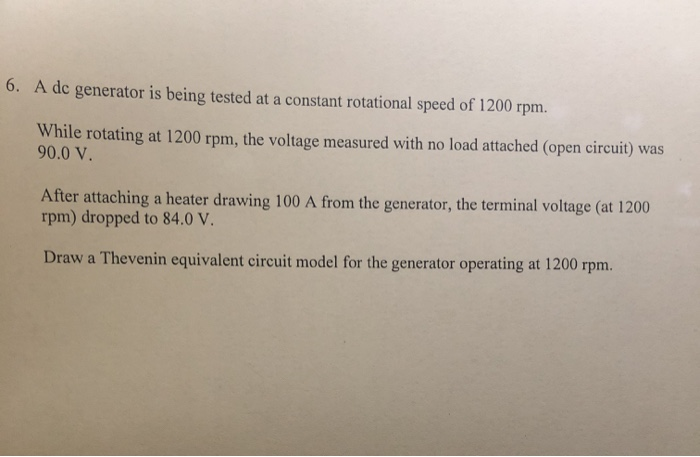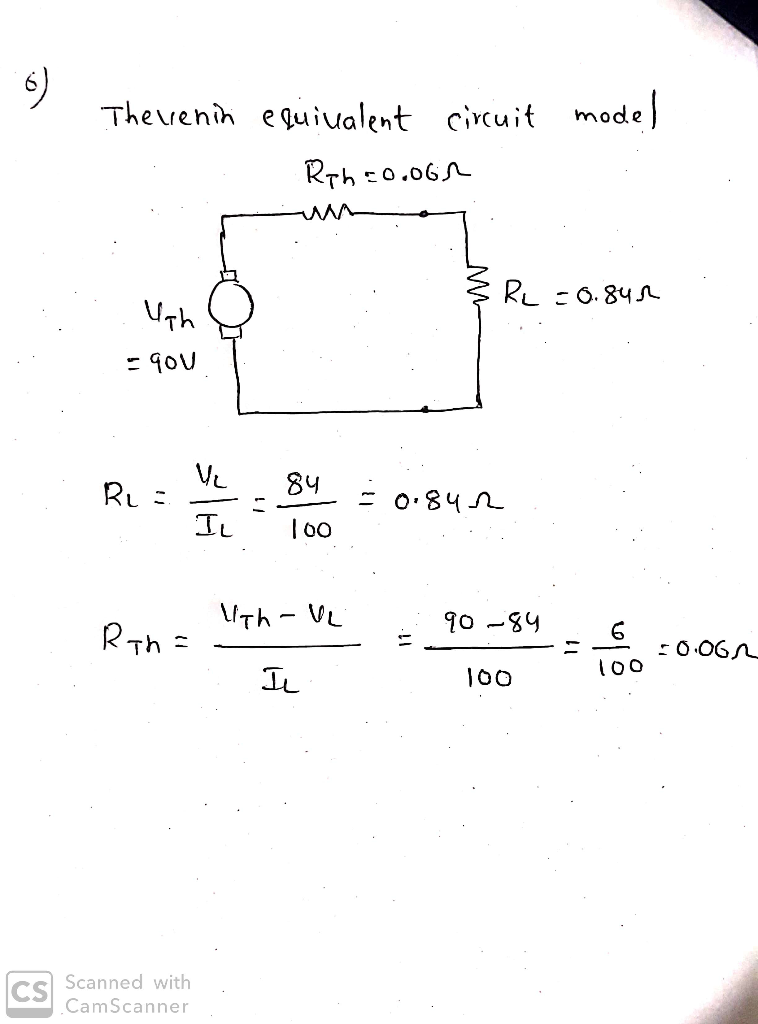#### Earn Coins

Coins can be redeemed for fabulous gifts.

Similar Homework Help Questions
• ### 2. A dc machine (6 kW, 120 V, 1200 rpm, Ra = 0.2 Ω, Rf =...

2. A dc machine (6 kW, 120 V, 1200 rpm, Ra = 0.2 Ω, Rf = 100 Ω) has the following open circuit characteristics at 1200 rpm. If (A) 0 0.1 0.2 0.3 0.4 0.5 0.6 0.8 1.0 1.2 1.4 Ea (V) 5 20 40 60 79 93 102 114 120 125 130 It has a field control resistance whose value can be varied from 0 to 150 Ω. The machine is driven at 1200 rpm and separately excited from...

• ### Q1: (30 points) A shunt DC generator, running at a constant speed of 1400 rpm, has...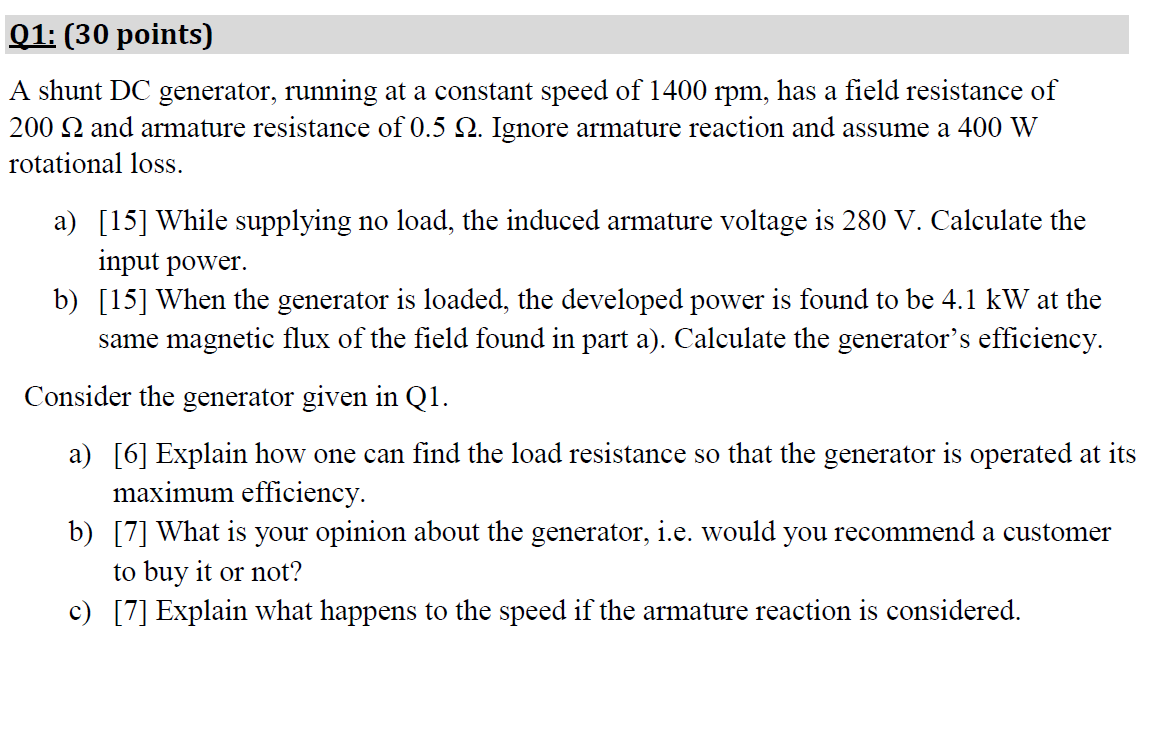Q1: (30 points) A shunt DC generator, running at a constant speed of 1400 rpm, has a field resistance of 200 22 and armature resistance of 0.5 12. Ignore armature reaction and assume a 400 W rotational loss. a)  While supplying no load, the induced armature voltage is 280 V. Calculate the input power. b)  When the generator is loaded, the developed power is found to be 4.1 kW at the same magnetic flux of the field found...

• ### A DC shunt generator delivers a load current of 50 A at 300 V with its...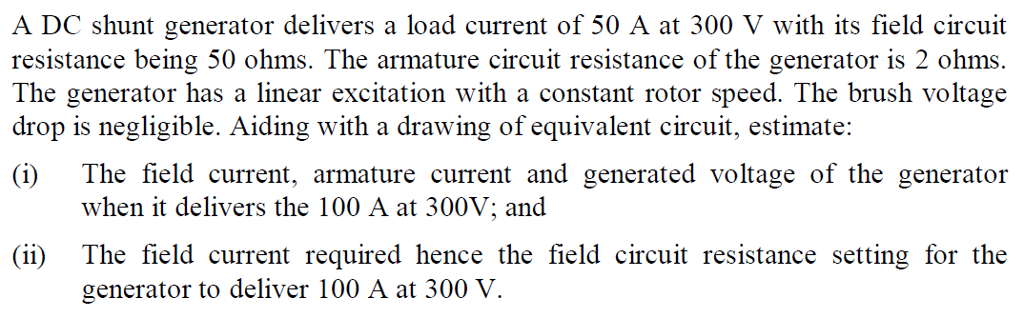A DC shunt generator delivers a load current of 50 A at 300 V with its field circuit resistance being 50 ohms. The armature circuit resistance of the generator is 2 ohms The generator has a linear excitation with a constant rotor speed. The brush voltage drop is negligible. Aiding with a drawing of equivalent circuit, estimate: i) The field current, armature current and generated voltage of the generator when it delivers the 100 A at 300V; and (ii) The...

• ### A 12 kV, 300 MVA synchronous generator is tested at a field current of 200 A....

A 12 kV, 300 MVA synchronous generator is tested at a field current of 200 A. The open-circuit voltage was found to be 10 kV and the short-circuit current was 7.5 kA. The stator resistance was measured at 0.1 ohms per phase. i) Find the value of the synchronous reactance in per-unit. [ 2 marks ] ii) The synchronous generator is delivering half rated output at a terminal voltage of 5% below rated voltage and a power factor of 0.7...

• ### QUESTION 1 A) (10 marks) A 10 kW, 250 V DC self-excited shunt generator, with an...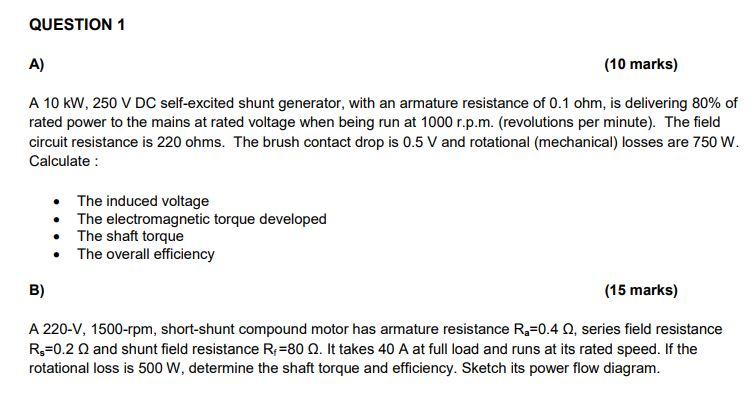QUESTION 1 A) (10 marks) A 10 kW, 250 V DC self-excited shunt generator, with an armature resistance of 0.1 ohm, is delivering 80 % of rated power to the mains at rated voltage when being run at 1000 r.p.m. (revolutions per minute). The field circuit resistance is 220 ohms. The brush contact drop is 0.5 V and rotational (mechanical) losses are 750 W Calculate The induced voltage The electromagnetic torque developed The shaft torque The overall efficiency B) (15...

• ### T u resistance in a DC is decreased while the motor is driving a ii. A...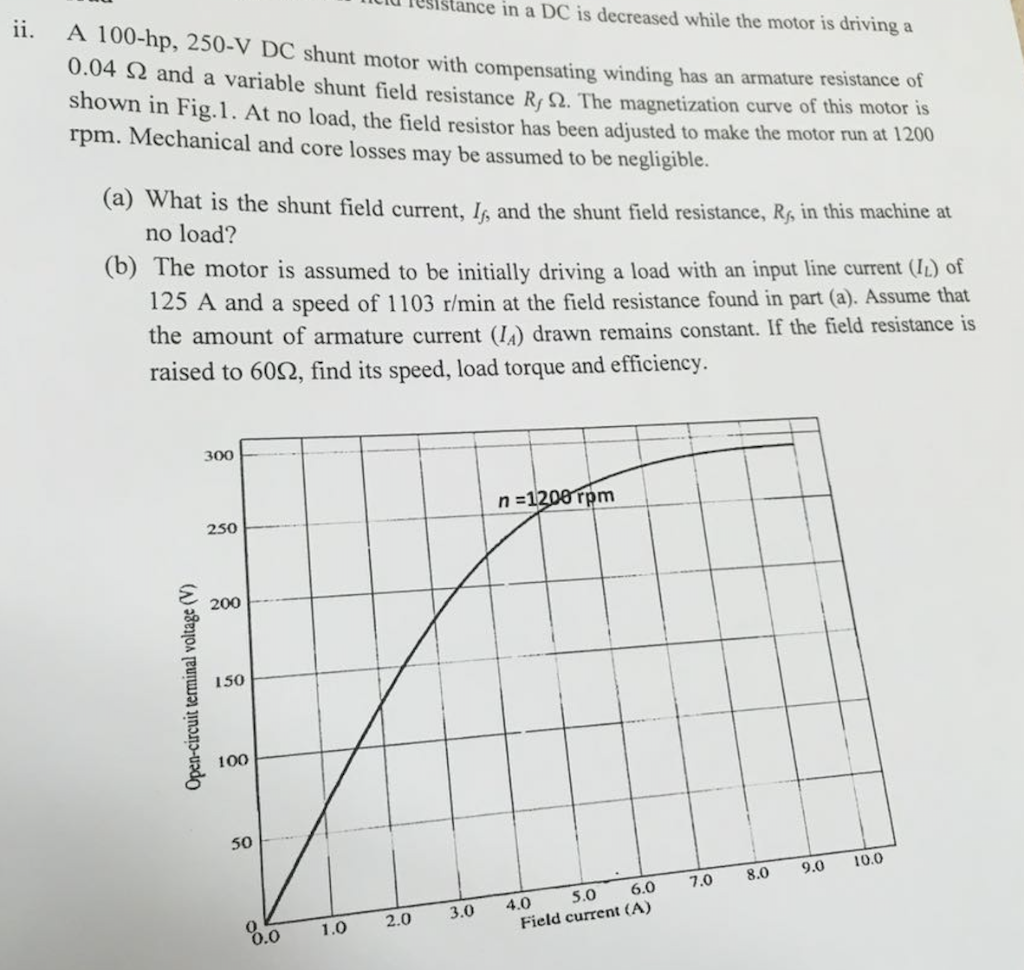T u resistance in a DC is decreased while the motor is driving a ii. A 100-hp, 250-V DC shunt motor with compensating winding has an am 0.04 12 and a variable shunt field resistance R; 92. The magn ompensating winding has an armature resistance of shunt field resistance R, 12. The magnetization curve of this motor is shown in Fig. 1. At no load, the field resistor has been a . At no load, the field resistor has been...

• ### EE 282-Circuit I Pre-Lab 9 Maximum Power Transfer Theorem Name Concepts: In this pre-lab we will ...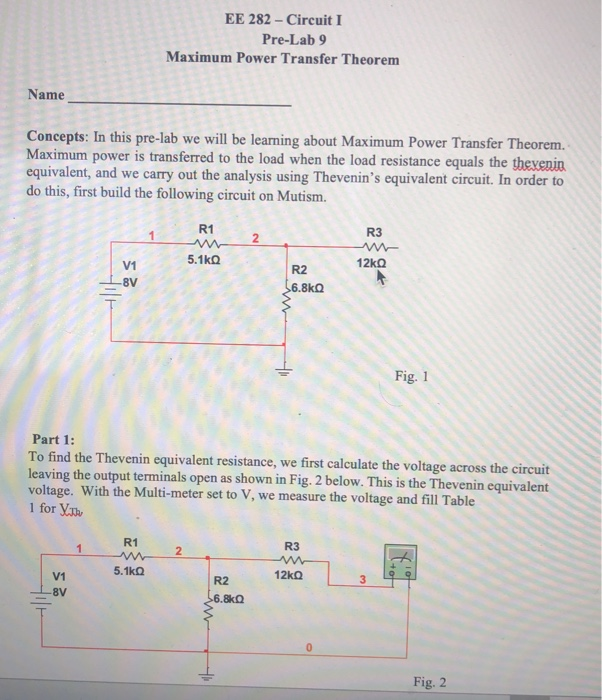EE 282-Circuit I Pre-Lab 9 Maximum Power Transfer Theorem Name Concepts: In this pre-lab we will be leaming about Maximum Power Transfer Theorem. Maximum power is transferred to the load when the load resistance equals the thexenin equivalent, and we carry out the analysis using Thevenin's equivalent circuit. In order to do this, first build the following circuit on Mutism. 1 R1 5.1k0 R3 2 V1 R2 8kQ 6.8㏀ Fig. 1 Part 1: To find the Thevenin equivalent resistance, we...

• ### summatize the following info and break them into differeng key points. write them in yojr own...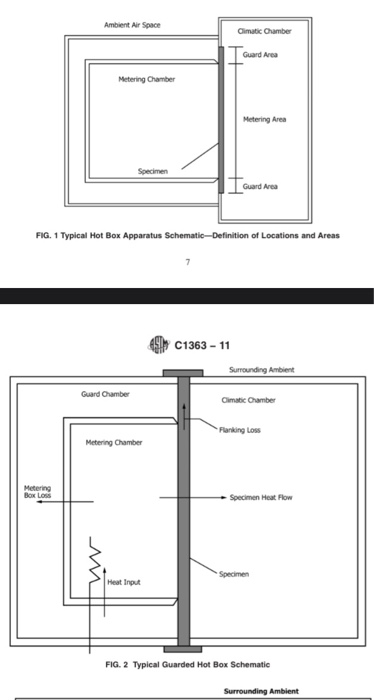summatize the following info and break them into differeng key points. write them in yojr own words   apartus 6.1 Introduction—The design of a successful hot box appa- ratus is influenced by many factors. Before beginning the design of an apparatus meeting this standard, the designer shall review the discussion on the limitations and accuracy, Section 13, discussions of the energy flows in a hot box, Annex A2, the metering box wall loss flow, Annex A3, and flanking loss, Annex...